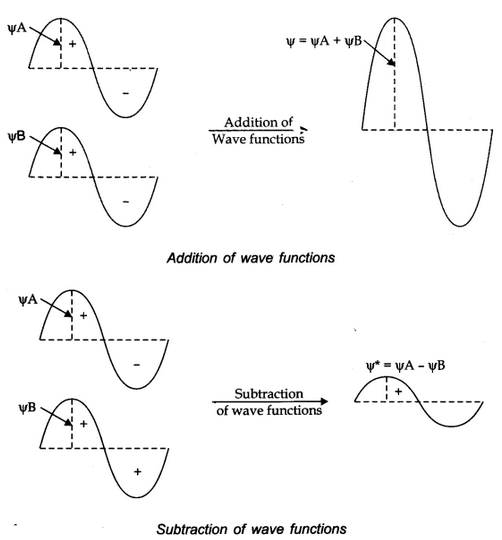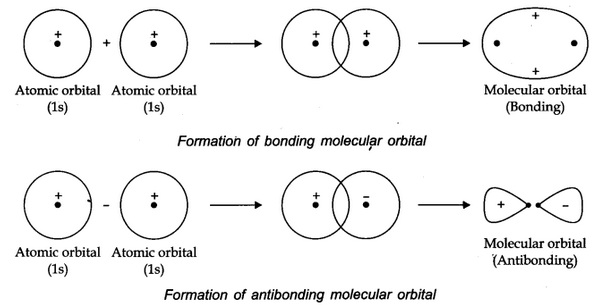# Molecular Orbital Theory

According to this theory, the atomic orbitals combine to form the molecular orbitals. The number of molecular orbitals formed is equal is the number of atomic orbitals involved. According to this theory.

# Linear Combination of Atomic Orbitals (LCAO)

The formation of molecular orbitals can be explained by the linear combination of atomic orbitals. Combination takes place either by addition or by subtraction of wave function as shown below.The molecular orbital formed by addition orbitals is called bonding molecular orbital while molecular orbital formed by subtraction of atomic orbitals is called antibonding molecular orbital.

## Conditions for the combination of atomic orbitals:

• The combining atomic orbitals must have almost equal energy.
• The combining atomic orbitals must have same symmetry about the molecular axis.
• The combining atomic orbitals must overlap to the maximum extent.

# Types of molecular Orbitals

## Sigma (σ) molecular Orbitals:

They are symmetrical around the bond-axis.

## Pi (π) Molecular Orbitals:

They are not symmetrical, because of the presence of positive lobes above and negative lobes below the molecular plane.

# Stability of Molecules

If Nb = No. of electrons occupying bonding orbitals.

Na = No. of electrons occupying antibonding orbitals.

then

• If Nb > Na molecular will be stable.
• Nb < Na molecular will be unstable